Rigid Body Dynamics Library
Constraints

## Data Structures

struct  ConstraintSet
Structure that contains both constraint information and workspace memory. More...

struct  CustomConstraint
Interface to define general-purpose constraints. More...

## Functions

RBDL_DLLAPI void CalcConstraintsPositionError (Model &model, const Math::VectorNd &Q, ConstraintSet &CS, Math::VectorNd &err, bool update_kinematics=true)
Computes the position errors for the given ConstraintSet. More...

RBDL_DLLAPI void CalcConstraintsJacobian (Model &model, const Math::VectorNd &Q, ConstraintSet &CS, Math::MatrixNd &G, bool update_kinematics=true)
Computes the Jacobian for the given ConstraintSet. More...

RBDL_DLLAPI void CalcConstraintsVelocityError (Model &model, const Math::VectorNd &Q, const Math::VectorNd &QDot, ConstraintSet &CS, Math::VectorNd &err, bool update_kinematics=true)
Computes the velocity errors for the given ConstraintSet. More...

RBDL_DLLAPI void CalcConstrainedSystemVariables (Model &model, const Math::VectorNd &Q, const Math::VectorNd &QDot, const Math::VectorNd &Tau, ConstraintSet &CS, std::vector< Math::SpatialVector > *f_ext=NULL)
Computes the terms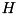,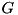, andof the constrained dynamic problem and stores them in the ConstraintSet. More...

RBDL_DLLAPI bool CalcAssemblyQ (Model &model, Math::VectorNd QInit, ConstraintSet &CS, Math::VectorNd &Q, const Math::VectorNd &weights, double tolerance=1e-12, unsigned int max_iter=100)
Computes a feasible initial value of the generalized joint positions. More...

RBDL_DLLAPI void CalcAssemblyQDot (Model &model, const Math::VectorNd &Q, const Math::VectorNd &QDotInit, ConstraintSet &CS, Math::VectorNd &QDot, const Math::VectorNd &weights)
Computes a feasible initial value of the generalized joint velocities. More...

RBDL_DLLAPI void ForwardDynamicsConstraintsDirect (Model &model, const Math::VectorNd &Q, const Math::VectorNd &QDot, const Math::VectorNd &Tau, ConstraintSet &CS, Math::VectorNd &QDDot, std::vector< Math::SpatialVector > *f_ext=NULL)
Computes forward dynamics with contact by constructing and solving the full lagrangian equation. More...

RBDL_DLLAPI void ForwardDynamicsConstraintsRangeSpaceSparse (Model &model, const Math::VectorNd &Q, const Math::VectorNd &QDot, const Math::VectorNd &Tau, ConstraintSet &CS, Math::VectorNd &QDDot, std::vector< Math::SpatialVector > *f_ext)

RBDL_DLLAPI void ForwardDynamicsConstraintsNullSpace (Model &model, const VectorNd &Q, const VectorNd &QDot, const VectorNd &Tau, ConstraintSet &CS, VectorNd &QDDot, std::vector< Math::SpatialVector > *f_ext)

RBDL_DLLAPI void ForwardDynamicsContactsKokkevis (Model &model, const Math::VectorNd &Q, const Math::VectorNd &QDot, const Math::VectorNd &Tau, ConstraintSet &CS, Math::VectorNd &QDDot)
Computes forward dynamics that accounts for active contacts in ConstraintSet. More...

RBDL_DLLAPI void ComputeConstraintImpulsesDirect (Model &model, const Math::VectorNd &Q, const Math::VectorNd &QDotMinus, ConstraintSet &CS, Math::VectorNd &QDotPlus)
Computes contact gain by constructing and solving the full lagrangian equation. More...

RBDL_DLLAPI void ComputeConstraintImpulsesRangeSpaceSparse (Model &model, const Math::VectorNd &Q, const Math::VectorNd &QDotMinus, ConstraintSet &CS, Math::VectorNd &QDotPlus)
Resolves contact gain using SolveContactSystemRangeSpaceSparse() More...

RBDL_DLLAPI void ComputeConstraintImpulsesNullSpace (Model &model, const Math::VectorNd &Q, const Math::VectorNd &QDotMinus, ConstraintSet &CS, Math::VectorNd &QDotPlus)
Resolves contact gain using SolveContactSystemNullSpace() More...

RBDL_DLLAPI void SolveConstrainedSystemDirect (Math::MatrixNd &H, const Math::MatrixNd &G, const Math::VectorNd &c, const Math::VectorNd &gamma, Math::VectorNd &qddot, Math::VectorNd &lambda, Math::MatrixNd &A, Math::VectorNd &b, Math::VectorNd &x, Math::LinearSolver &linear_solver)
Solves the full contact system directly, i.e. simultaneously for contact forces and joint accelerations. More...

RBDL_DLLAPI void SolveConstrainedSystemRangeSpaceSparse (Model &model, Math::MatrixNd &H, const Math::MatrixNd &G, const Math::VectorNd &c, const Math::VectorNd &gamma, Math::VectorNd &qddot, Math::VectorNd &lambda, Math::MatrixNd &K, Math::VectorNd &a, Math::LinearSolver linear_solver)
Solves the contact system by first solving for the the joint accelerations and then the contact forces using a sparse matrix decomposition of the joint space inertia matrix. More...

RBDL_DLLAPI void SolveConstrainedSystemNullSpace (Math::MatrixNd &H, const Math::MatrixNd &G, const Math::VectorNd &c, const Math::VectorNd &gamma, Math::VectorNd &qddot, Math::VectorNd &lambda, Math::MatrixNd &Y, Math::MatrixNd &Z, Math::VectorNd &qddot_y, Math::VectorNd &qddot_z, Math::LinearSolver &linear_solver)
Solves the contact system by first solving for the joint accelerations and then for the constraint forces. More...

## Variables

struct RBDL_DLLAPI CustomConstraint

## Detailed Description

Constraints are handled by specification of a ConstraintSet which contains all informations about the current constraints and workspace memory.

Separate constraints can be specified by calling ConstraintSet::AddContactConstraint(), ConstraintSet::AddLoopConstraint(), and ConstraintSet::AddCustomConstraint(). After all constraints have been specified, this ConstraintSet has to be bound to the model via ConstraintSet::Bind(). This initializes workspace memory that is later used when calling one of the contact functions, such as ForwardDynamicsContactsDirects().

The values in the vectors ConstraintSet::force and ConstraintSet::impulse contain the computed force or impulse values for each constraint when returning from one of the contact functions.

# Solution of the Constraint System

## Linear System of the Constrained Dynamics

In the presence of constraints, to compute the acceleration one has to solve a linear system of the form: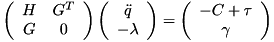whereis the joint space inertia matrix computed with the CompositeRigidBodyAlgorithm(),is the constraint jacobian,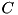the bias force (sometimes called "non-linear effects"), andthe generalized acceleration independent part of the constraints.

## Linear System of the Contact Collision

Similarly to compute the response of the model to a contact gain one has to solve a system of the following form: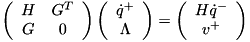whereis the joint space inertia matrix computed with the CompositeRigidBodyAlgorithm(),are the point jacobians of the contact points,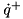the generalized velocity after the impact,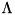the impulses at each constraint,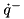the generalized velocity before the impact, and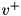the desired velocity of each constraint after the impact (known beforehand, usually 0). The value ofcan is specified via the variable ConstraintSet::v_plus and defaults to 0.

## Solution Methods

There are essentially three different approaches to solve these systems:

1. Direct: solve the full system to simultaneously compute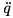and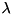. This may be slow for large systems and many constraints.
2. Range-Space: solve first forand then for.
3. Null-Space: solve furst forand then forThe methods are the same for the contact gains just with different variables on the right-hand-side.

RBDL provides methods for all approaches. The implementation for the range-space method also exploits sparsities in the joint space inertia matrix using a sparse structure preserving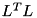decomposition as described in Chapter 8.5 of "Rigid Body Dynamics Algorithms".

None of the methods is generally superior to the others and each has different trade-offs as factors such as model topology, number of constraints, constrained bodies, numerical stability, and performance vary and evaluation has to be made on a case-by-case basis.

## Methods for Solving Constrained

Dynamics

RBDL provides the following methods to compute the acceleration of a constrained system:

## Methods for Computing Collisions

RBDL provides the following methods to compute the collision response on new contact gains:

## Computing generalized joint positions and velocities

satisfying the constraint equations.

When considering a model subject position level constraints expressed by the equation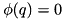, it is often necessary to compute generalized joint position and velocities which satisfy the constraints. Even velocity-level constraints may have position-level assembly constraints: a rolling-without-slipping constraint is a velocity-level constraint, but during assembly it might be desireable to put the rolling surfaces in contact with eachother.

In order to compute a vector of generalized joint positions that satisfy the constraints it is necessary to solve the following optimization problem:In order to compute a vector of generalized joint velocities that satisfy the constraints it is necessary to solve the following optimization problem: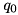and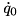are initial guesses,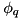is the constraints jacobian (partial derivative of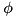with respect to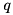), and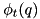is the partial derivative ofwith respect to time.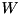is a diagonal weighting matrix, which can be exploited to prioritize changes in the position/ velocity of specific joints. With a solver capable of handling singular matrices, it is possible to set to 1 the weight of the joint positions/ velocities that should not be changed from the initial guesses, and to 0 those corresponding to the values that can be changed.

These problems are solved using the Lagrange multipliers method. For the velocity problem the solution is exact. For the position problem the constraints are linearized in the form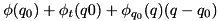and the linearized problem is solved iteratively until the constraint position errors are smaller than a given threshold.

RBDL provides two functions to compute feasible joint position and velocities:

## Baumgarte Stabilization

The constrained dynamic equations are correct at the acceleration level but will drift at the velocity and position level during numerical integration. RBDL implements Baumgarte stabilization to avoid the accumulation of position and velocity errors for loop constraints and custom constraints. Contact constraints do not have Baumgarte stabilization because they are a special case which does not typically suffer from drift. The stabilization term can be enabled/disabled using the appropriate ConstraintSet::AddLoopConstraint and ConstraintSet::AddCustomConstraint functions.

The dynamic equations are changed to the following form: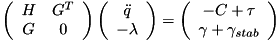A term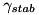is added to the right hand side of the equation, defined in the following way: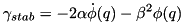where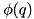are the position level constraint errors and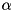and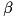are tuning coefficients. There is no clear and simple rule on how to choose them as good values also depend on the used integration method and time step. If the values are too small the constrained dynamics equation becomes stiff, too big values result in errors not being reduced.

A good starting point is to parameterize the coefficients as: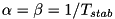with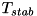specifies a time constant for errors in position and velocity errors to reduce. Featherstone suggests in his book "Rigid Body Dynamics Algorithms" that for a big industrial robot a value of 0.1 is reasonable. When testing different values best is to try different orders of magnitude as e.g. doubling a value only has little effect.

For Loop- and CustomConstraints Baumgarte stabilization is enabled by default and uses the stabilization parameter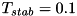.

## ◆ CalcAssemblyQ()

 RBDL_DLLAPI bool CalcAssemblyQ ( Model & model, Math::VectorNd QInit, ConstraintSet & CS, Math::VectorNd & Q, const Math::VectorNd & weights, double tolerance = 1e-12, unsigned int max_iter = 100 )

Computes a feasible initial value of the generalized joint positions.

Parameters
 model the model QInit initial guess for the generalized positions of the joints CS the constraint set for which the error should be computed Q (output) vector of the generalized joint positions. weights weighting coefficients for the different joint positions. tolerance the function will return successfully if the constraint position error norm is lower than this value. max_iter the funciton will return unsuccessfully after performing this number of iterations.
Returns
true if the generalized joint positions were computed successfully, false otherwise.

Definition at line 819 of file Constraints.cc.

## ◆ CalcAssemblyQDot()

 RBDL_DLLAPI void CalcAssemblyQDot ( Model & model, const Math::VectorNd & Q, const Math::VectorNd & QDotInit, ConstraintSet & CS, Math::VectorNd & QDot, const Math::VectorNd & weights )

Computes a feasible initial value of the generalized joint velocities.

Parameters
 model the model Q the generalized joint position of the joints. It is assumed that this vector satisfies the position level assemblt constraints. QDotInit initial guess for the generalized velocities of the joints CS the constraint set for which the error should be computed QDot (output) vector of the generalized joint velocities. weights weighting coefficients for the different joint positions.

Definition at line 926 of file Constraints.cc.

## ◆ CalcConstrainedSystemVariables()

 RBDL_DLLAPI void CalcConstrainedSystemVariables ( Model & model, const Math::VectorNd & Q, const Math::VectorNd & QDot, const Math::VectorNd & Tau, ConstraintSet & CS, std::vector< Math::SpatialVector > * f_ext = NULL )

Computes the terms,, andof the constrained dynamic problem and stores them in the ConstraintSet.

Parameters
 model the model Q the generalized positions of the joints QDot the generalized velocities of the joints Tau the generalized forces of the joints CS the constraint set for which the error should be computed f_ext External forces acting on the body in base coordinates (optional, defaults to NULL)
Note
This function is normally called automatically in the various constrained dynamics functions, the user normally does not have to call it.

Definition at line 696 of file Constraints.cc.

## ◆ CalcConstraintsJacobian()

 RBDL_DLLAPI void CalcConstraintsJacobian ( Model & model, const Math::VectorNd & Q, ConstraintSet & CS, Math::MatrixNd & G, bool update_kinematics = true )

Computes the Jacobian for the given ConstraintSet.

Parameters
 model the model Q the generalized positions of the joints CS the constraint set for which the Jacobian should be computed G (output) matrix where the output will be stored in update_kinematics whether the kinematics of the model should be updated from Q

Definition at line 570 of file Constraints.cc.

## ◆ CalcConstraintsPositionError()

 RBDL_DLLAPI void CalcConstraintsPositionError ( Model & model, const Math::VectorNd & Q, ConstraintSet & CS, Math::VectorNd & err, bool update_kinematics = true )

Computes the position errors for the given ConstraintSet.

Parameters
 model the model Q the generalized positions of the joints CS the constraint set for which the error should be computed err (output) vector where the error will be stored in (should have the size of CS). update_kinematics whether the kinematics of the model should be updated from Q.
Note
the position error is always 0 for contact constraints.

Definition at line 498 of file Constraints.cc.

## ◆ CalcConstraintsVelocityError()

 RBDL_DLLAPI void CalcConstraintsVelocityError ( Model & model, const Math::VectorNd & Q, const Math::VectorNd & QDot, ConstraintSet & CS, Math::VectorNd & err, bool update_kinematics = true )

Computes the velocity errors for the given ConstraintSet.

Parameters
 model the model Q the generalized positions of the joints QDot the generalized velocities of the joints CS the constraint set for which the error should be computed err (output) vector where the error will be stored in (should have the size of CS). update_kinematics whether the kinematics of the model should be updated from Q.
Note
this is equivalent to multiplying the constraint jacobian by the generalized velocities of the joints.

Definition at line 666 of file Constraints.cc.

## ◆ ComputeConstraintImpulsesDirect()

 RBDL_DLLAPI void ComputeConstraintImpulsesDirect ( Model & model, const Math::VectorNd & Q, const Math::VectorNd & QDotMinus, ConstraintSet & CS, Math::VectorNd & QDotPlus )

Computes contact gain by constructing and solving the full lagrangian equation.

This method builds and solves the linear systemwhereis the joint space inertia matrix computed with the CompositeRigidBodyAlgorithm(),are the point jacobians of the contact points,the generalized velocity after the impact,the impulses at each constraint,the generalized velocity before the impact, andthe desired velocity of each constraint after the impact (known beforehand, usually 0). The value ofcan is specified via the variable ConstraintSet::v_plus and defaults to 0.

Note
So far, only constraints acting along cartesian coordinate axes are allowed (i.e. (1, 0, 0), (0, 1, 0), and (0, 0, 1)). Also, one must not specify redundant constraints!
Note
To increase performance group constraints body and pointwise such that constraints acting on the same body point are sequentially in ConstraintSet. This can save computation of point Jacobians.
Parameters
 model rigid body model Q state vector of the internal joints QDotMinus velocity vector of the internal joints before the impact CS the set of active constraints QDotPlus velocities of the internals joints after the impact (output)

Definition at line 1053 of file Constraints.cc.

## ◆ ComputeConstraintImpulsesNullSpace()

 RBDL_DLLAPI void ComputeConstraintImpulsesNullSpace ( Model & model, const Math::VectorNd & Q, const Math::VectorNd & QDotMinus, ConstraintSet & CS, Math::VectorNd & QDotPlus )

Resolves contact gain using SolveContactSystemNullSpace()

Definition at line 1104 of file Constraints.cc.

## ◆ ComputeConstraintImpulsesRangeSpaceSparse()

 RBDL_DLLAPI void ComputeConstraintImpulsesRangeSpaceSparse ( Model & model, const Math::VectorNd & Q, const Math::VectorNd & QDotMinus, ConstraintSet & CS, Math::VectorNd & QDotPlus )

Resolves contact gain using SolveContactSystemRangeSpaceSparse()

Definition at line 1083 of file Constraints.cc.

## ◆ ForwardDynamicsConstraintsDirect()

 RBDL_DLLAPI void ForwardDynamicsConstraintsDirect ( Model & model, const Math::VectorNd & Q, const Math::VectorNd & QDot, const Math::VectorNd & Tau, ConstraintSet & CS, Math::VectorNd & QDDot, std::vector< Math::SpatialVector > * f_ext = NULL )

Computes forward dynamics with contact by constructing and solving the full lagrangian equation.

This method builds and solves the linear systemwhereis the joint space inertia matrix computed with the CompositeRigidBodyAlgorithm(),are the point jacobians of the contact points,the bias force (sometimes called "non-linear effects"), andthe generalized acceleration independent part of the contact point accelerations.

Note
This function works with ContactConstraints, LoopConstraints and Custom Constraints. Nonetheless, this method will not tolerate redundant constraints.
Note
To increase performance group constraints body and pointwise such that constraints acting on the same body point are sequentially in ConstraintSet. This can save computation of point jacobians.
Parameters
 model rigid body model Q state vector of the internal joints QDot velocity vector of the internal joints Tau actuations of the internal joints CS the description of all acting constraints QDDot accelerations of the internals joints (output) f_ext External forces acting on the body in base coordinates (optional, defaults to NULL)
Note
During execution of this function values such as ConstraintSet::force get modified and will contain the value of the force acting along the normal.

Definition at line 980 of file Constraints.cc.

## ◆ ForwardDynamicsConstraintsNullSpace()

 RBDL_DLLAPI void ForwardDynamicsConstraintsNullSpace ( Model & model, const VectorNd & Q, const VectorNd & QDot, const VectorNd & Tau, ConstraintSet & CS, VectorNd & QDDot, std::vector< Math::SpatialVector > * f_ext )

## ◆ ForwardDynamicsConstraintsRangeSpaceSparse()

 RBDL_DLLAPI void ForwardDynamicsConstraintsRangeSpaceSparse ( Model & model, const Math::VectorNd & Q, const Math::VectorNd & QDot, const Math::VectorNd & Tau, ConstraintSet & CS, Math::VectorNd & QDDot, std::vector< Math::SpatialVector > * f_ext )

## ◆ ForwardDynamicsContactsKokkevis()

 RBDL_DLLAPI void ForwardDynamicsContactsKokkevis ( Model & model, const Math::VectorNd & Q, const Math::VectorNd & QDot, const Math::VectorNd & Tau, ConstraintSet & CS, Math::VectorNd & QDDot )

Computes forward dynamics that accounts for active contacts in ConstraintSet.

The method used here is the one described by Kokkevis and Metaxas in the Paper "Practical Physics for Articulated Characters", Game Developers Conference, 2004.

It does this by recursively computing the inverse articulated-body inertia (IABI)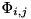which is then used to build and solve a system of the form:Here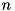is the number of constraints and the method for building the system uses the Articulated Body Algorithm to efficiently compute entries of the system. The values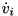are the constraint accelerations,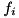the constraint forces, and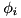are the constraint bias forces.

Parameters
 model rigid body model Q state vector of the internal joints QDot velocity vector of the internal joints Tau actuations of the internal joints CS a list of all contact points QDDot accelerations of the internals joints (output)
Note
During execution of this function values such as ConstraintSet::force get modified and will contain the value of the force acting along the normal.
This function supports only contact constraints.
Todo:
Allow for external forces

Definition at line 1445 of file Constraints.cc.

## ◆ SolveConstrainedSystemDirect()

 RBDL_DLLAPI void SolveConstrainedSystemDirect ( Math::MatrixNd & H, const Math::MatrixNd & G, const Math::VectorNd & c, const Math::VectorNd & gamma, Math::VectorNd & qddot, Math::VectorNd & lambda, Math::MatrixNd & A, Math::VectorNd & b, Math::VectorNd & x, Math::LinearSolver & linear_solver )

Solves the full contact system directly, i.e. simultaneously for contact forces and joint accelerations.

This solves a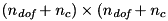linear system.

Parameters
 H the joint space inertia matrix G the constraint jacobian c the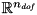vector of the upper part of the right hand side of the system gamma the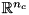vector of the lower part of the right hand side of the system qddot result: joint accelerations lambda result: constraint forces A work-space for the matrix of the linear system b work-space for the right-hand-side of the linear system x work-space for the solution of the linear system type of solver that should be used to solve the system

Definition at line 349 of file Constraints.cc.

## ◆ SolveConstrainedSystemNullSpace()

 RBDL_DLLAPI void SolveConstrainedSystemNullSpace ( Math::MatrixNd & H, const Math::MatrixNd & G, const Math::VectorNd & c, const Math::VectorNd & gamma, Math::VectorNd & qddot, Math::VectorNd & lambda, Math::MatrixNd & Y, Math::MatrixNd & Z, Math::VectorNd & qddot_y, Math::VectorNd & qddot_z, Math::LinearSolver & linear_solver )

Solves the contact system by first solving for the joint accelerations and then for the constraint forces.

This methods requires a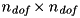matrix of the form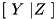with the property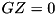that can be computed using a QR decomposition (e.g. see code for ForwardDynamicsContactsNullSpace()).

Parameters
 H the joint space inertia matrix G the constraint jacobian c thevector of the upper part of the right hand side of the system gamma thevector of the lower part of the right hand side of the system qddot result: joint accelerations lambda result: constraint forces Y basis for the range-space of the constraints Z basis for the null-space of the constraints qddot_y work-space of sizeqddot_z work-space of sizelinear_solver type of solver that should be used to solve the system

Definition at line 437 of file Constraints.cc.

## ◆ SolveConstrainedSystemRangeSpaceSparse()

 RBDL_DLLAPI void SolveConstrainedSystemRangeSpaceSparse ( Model & model, Math::MatrixNd & H, const Math::MatrixNd & G, const Math::VectorNd & c, const Math::VectorNd & gamma, Math::VectorNd & qddot, Math::VectorNd & lambda, Math::MatrixNd & K, Math::VectorNd & a, Math::LinearSolver linear_solver )

Solves the contact system by first solving for the the joint accelerations and then the contact forces using a sparse matrix decomposition of the joint space inertia matrix.

This method exploits the branch-induced sparsity by the structure preservingdecomposition described in RBDL, Section 6.5.

Parameters
 H the joint space inertia matrix G the constraint jacobian c thevector of the upper part of the right hand side of the system gamma thevector of the lower part of the right hand side of the system qddot result: joint accelerations lambda result: constraint forces K work-space for the matrix of the constraint force linear system a work-space for the right-hand-side of the constraint force linear system linear_solver type of solver that should be used to solve the constraint force system

Definition at line 400 of file Constraints.cc.

## ◆ CustomConstraint

 struct RBDL_DLLAPI CustomConstraint

Definition at line 255 of file Constraints.h.